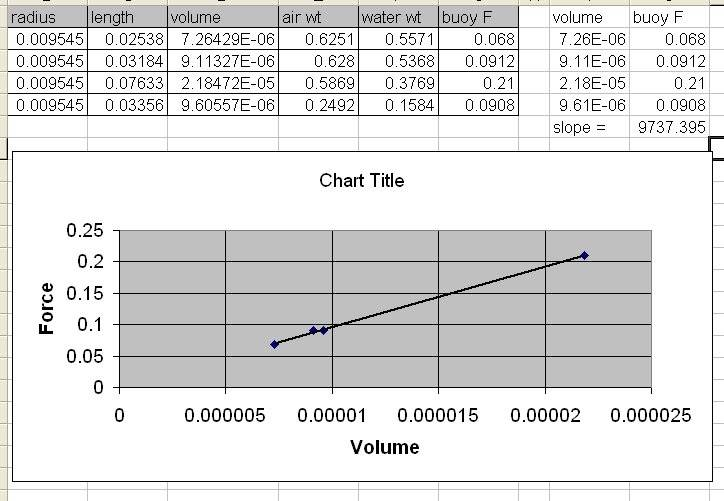# Determining the Density of Water

1. In this experiment, which we did in lab, we measured the diameter and length of 4 metal cylinders of different densities. These cylinders were hung on a string and the force in Newtons was measured. Then the cylinder was submerged in water and the force was once again taken. These values were used to calculate the buoyant force. We were then supposed to graph the Buoyant Force vs the Volume. We entered these into the Graphical Analysis program and found the best fit line, the slope of which should have been ρg, or the density of water times g. This is where we encountered the problem. Our slope was way off.

2. Homework Equations :
Volume of a cylinder: V = L∏r^2 (length*pi*radius squared)
Weight of cylinder in air: F$_{A}$ = mg
Weight in water: F$_{W}$ = mg - F$_{B}$
Buoyant Force: F$_{B}$ = F$_{A}$ - F$_{W}$

Diameters for the cylinders:
1.) 1.909 * 10$^{-2}$ meters
2.) 1.902 * 10$^{-2}$ meters
3.) 1.909 * 10$^{-2}$ meters
4.) 1.904 * 10$^{-2}$ meters

Lengths of the cylinders:
1.) 2.538 * 10$^{-2}$ meters
2.) 3.184 * 10$^{-2}$ meters
3.) 7.633 * 10$^{-2}$ meters
4.) 3.356 * 10$^{-2}$ meters

The volumes as I calculate them to be:
1.) 7.264 * 10$^{-6}$ meters cubed
2.) 9.047 * 10$^{-6}$ meters cubed
3.) 2.185 * 10$^{-5}$ meters cubed
4.) 9.555 * 10$^{-6}$ meters cubed

Weight in air:
1.) 0.6251 N
2.) 0.6280 N
3.) 0.5869 N
4.) 0.2492 N

Weight in water:
1.) 0.5571 N
2.) 0.5368 N
3.) 0.3769 N
4.) 0.1584 N

The buoyant forces are therefore:
1.) 0.0680 N
2.) 0.0912 N
3.) 0.2100 N
4.) 0.0908 N

When I plot F$_{B}$ vs Volume and find the best fit line, the slope is 7.56. Cylinder 3 seems to be the outlier. However, even if I take it out of the graph, the slope is 85.8. From what I gather, it should be somewhere near 998. I'm not sure why it isn't. The forces and volumes are, as far as I can tell, correct. I even looked up the density of the different metals in order to make sure that the weight out of water was close to what it should be. Cylinder 1 is copper, #2 is zinc, and #4 is aluminum. I'm not sure what #3 was, as it wasn't marked. Hope all of this is clear. I'm not sure what went wrong unless the equipment wasn't actually measuring in Newtons or it was measuring kiloNewtons or something weird like that. Help is much appreciated. Thanks in advance! :-)

Oh yes. I did make sure to divide the diameters by 2 in order to get the radii before calculating the volume, so those (I think) should be correct.

Delphi51
Homework Helper
I seem to be getting different answers for the volume. For the first one,
V = πr²h = π*.01909²*.02538 = 2.91 x 10^-5

We measured the diameters. I don't think you divided them by two in order to get the radius. In the example you used, .01909^2 should have been (0.01909/2)^2, or (0.009545)^2. Does that cause you to get the same volumes?

Delphi51
Homework Helper
I ran it all through this timeThe line of best fit seems to go through the first and third data points, so I used them to estimate the slope and got 9700. The density of water is 1000 kg/m3, so ρg should be about 9800. I hope you can spot the difference between your calc and mine so we can nail it!

Figured it out. Turns out, our Graphical Analysis program doesn't like it when you enter scientific notion in the wrong way and does weird things. On a sideish note, we checked all of the volume and buoyant force values to make sure there weren't any outliers. Since $\rho$g = ΔF/V (ΔF is the buoyant force), we were able to check the values we got for the buoyant force and volume to see if they were approximately equal to ρg.

First cylinder = (0.0680 N)/(7.264e-6 m$^{3}$) ≈ 9361
Second cylinder = (0.0912 N)/(9.047e-6 m$^{3}$ ≈ 10080
Third cylinder = (0.2100 N)/(2.185e-5 m$^{3}$) ≈ 9610
Fourth cylinder = (0.0908 N)/(9.555e-6 m$^{3}$) ≈ 9502

These are all pretty close to what you would expect to get if g = 9.8 m/s$^{2}$ and ρ of water = 1000 kg/m$^{3}$. ρg would be 9800.

Percent differences between experimental and calculated values of ρg
1.) 4.58%
2.) 2.82%
3.) 1.96%
4.) 3.09%

There were no outliers and the percent differences were relatively small. The issue came in with the program itself, not our data or calculations, which is good to know. Thanks for your help!

Delphi51
Homework Helper
Great!
This idea of calculating the difference between the "known" and experimental values is somewhat unsatisfying. I mean, if you "know" already, what is the point of doing the experiment again? The approach to error calcs that I like best is somehow estimating the accuracy of the measurements - may repeating some to see the variation, estimating instrument accuracy, etc. Then you use that knowledge to put error bars on the graph points. Finally, if the line of best fit actually fits within the error bars, you can say "The formula F = ρgV fits the data to within experimental error." This approach actually helps you discover new things.